# Distinct Subsequences| (DP-32)

Problem Statement: Distinct Subsequences

Problem Link: Subsequence Counting

We are given two strings S1 and S2, we want to know how many distinct subsequences of S2 are present in S1.

```Example: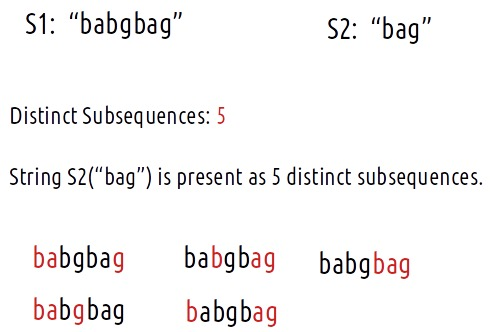```

Disclaimer: Don’t jump directly to the solution, try it out yourself first.

### Solution :

We have to find distinct subsequences of S2 in S1. As there is no uniformity in data, there is no other way to find out than to try out all possible ways. To do so we will need to use recursion.

Steps to form the recursive solution:

We will first form the recursive solution by the three points mentioned in the Dynamic Programming Introduction

Step 1: Express the problem in terms of indexes.

We are given two strings. We can represent them with the help of two indexes i and j. Initially, i=n-1 and j=m-1, where n and m are lengths of strings S1 and S2. Initially, we will call f(n-1,j-1), which means the count of all subsequences of string S2[0…m-1] in string S1[0…n-1]. We can generalize it as follows:Step 2: Try out all possible choices at a given index.

Now, i and j represent two characters from strings S1 and S2 respectively. We want to find distinct subsequences. There are only two options that make sense: either the characters represented by i and j match or they don’t.

Case 1: When the characters match

if(S1[i]==S2[j]), let’s understand it with the following example: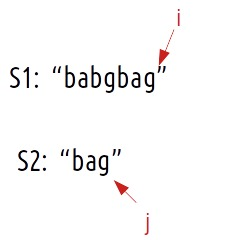S1[i] == S2[j], now as the characters at i and j match, we would want to check the possibility of the remaining characters of S2 in S1 therefore we reduce the length of both the strings by 1 and call the function recursively.Now, if we only make the above single recursive call, we are rejecting the opportunities to find more than one subsequences because it can happen that the jth character may match with more characters in S1[0…i-1], for example where there are more occurrences of ‘g’ in S1 from which also an answer needs to be explored.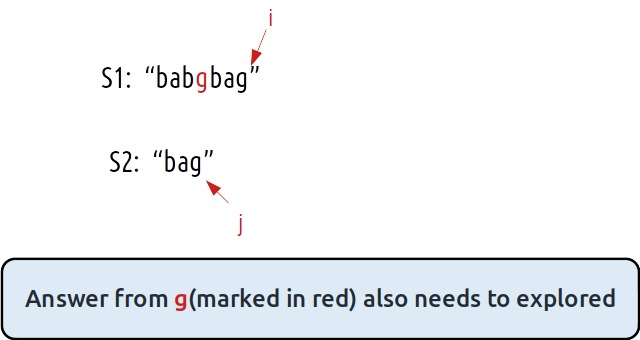To explore all such possibilities, we make another recursive call in which we reduce the length of the S1 string by 1 but keep the S2 string the same, i.e we call f(i-1,j).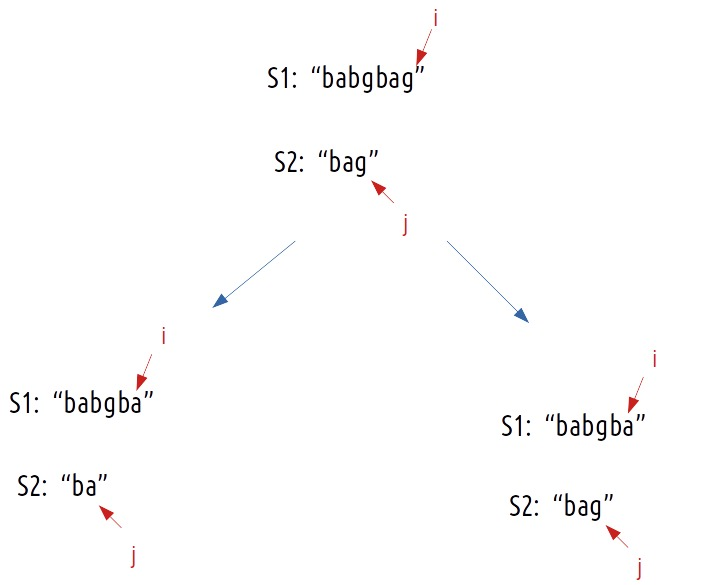Case 2: When the characters don’t match

if(S1[i] != S2[j]), it means that we don’t have any other choice than to try the next character of S1 and match it with the current character S2.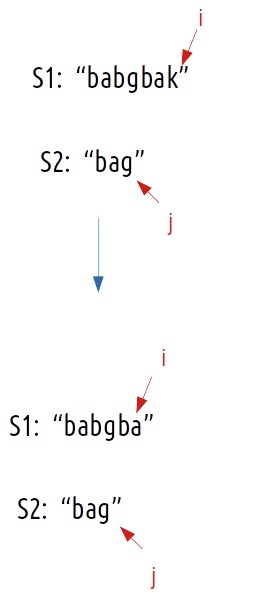This can be summarized as :

• if(S1[i]==S2[j]), call f(i-1,j-1) and f(i-1,j).
• if(S1[i]!=S2[j]), call f(i-1,j).

Step 3:  Return the sum of choices

As we have to return the total count, we will return the sum of f(i-1,j-1) and f(i-1,j) in case 1 and simply return f(i-1,j) in case 2.

Base Cases:

We are reducing i and j in our recursive relation, there can be two possibilities, either i becomes -1 or j becomes -1.

• if j<0, it means we have matched all characters of S2 with characters of S1, so we return 1.
• if i<0, it means we have checked all characters of S1 but we are not able to match all characters of S2, therefore we return 0.

The final pseudocode after steps 1, 2, and 3: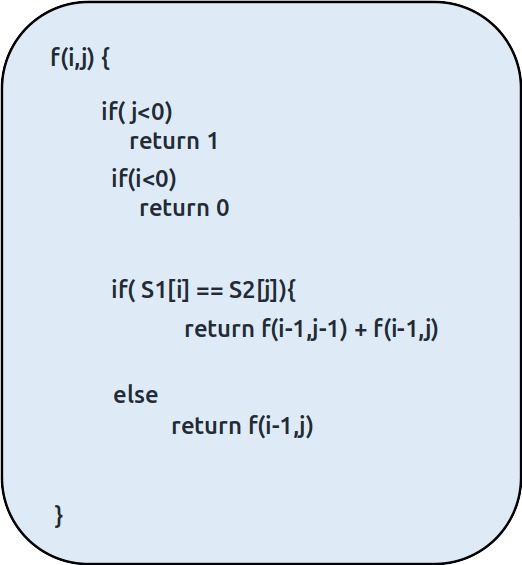Steps to memoize a recursive solution:

If we draw the recursion tree, we will see that there are overlapping subproblems. In order to convert a recursive solution the following steps will be taken:

1. Create a dp array of size [n][m]. The size of S1 and S2 are n and m respectively, so the variable i will always lie between ‘0’ and ‘n-1’ and the variable j between ‘0’ and ‘m-1’.
2. We initialize the dp array to -1.
3. Whenever we want to find the answer to particular parameters (say f(i,j)), we first check whether the answer is already calculated using the dp array(i.e dp[i][j]!= -1 ). If yes, simply return the value from the dp array.
4. If not, then we are finding the answer for the given value for the first time, we will use the recursive relation as usual but before returning from the function, we will set dp[i][j] to the solution we get.

Code:

## C++Code

``````#include <bits/stdc++.h>

using namespace std;

int prime = 1e9+7;

int countUtil(string s1, string s2, int ind1, int ind2,vector<vector<int>>& dp){
if(ind2<0)
return 1;
if(ind1<0)
return 0;

if(dp[ind1][ind2]!=-1)
return dp[ind1][ind2];

if(s1[ind1]==s2[ind2]){
int leaveOne = countUtil(s1,s2,ind1-1,ind2-1,dp);
int stay = countUtil(s1,s2,ind1-1,ind2,dp);

return dp[ind1][ind2] = (leaveOne + stay)%prime;
}

else{
return dp[ind1][ind2] = countUtil(s1,s2,ind1-1,ind2,dp);
}
}

int subsequenceCounting(string &t, string &s, int lt, int ls) {
// Write your code here.

vector<vector<int>> dp(lt,vector<int>(ls,-1));
return countUtil(t,s,lt-1,ls-1,dp);
}

int main() {

string s1 = "babgbag";
string s2 = "bag";

cout << "The Count of Distinct Subsequences is "
<<subsequenceCounting(s1,s2,s1.size(),s2.size());
}
``````

Output:

The Count of Distinct Subsequences is 5

Time Complexity: O(N*M)

Reason: There are N*M states therefore at max ‘N*M’ new problems will be solved.

Space Complexity: O(N*M) + O(N+M)

Reason: We are using a recursion stack space(O(N+M)) and a 2D array ( O(N*M)).

## Java Code

``````import java.util.*;

class TUF{
static int prime = (int)(Math.pow(10,9)+7);

static int countUtil(String s1, String s2, int ind1, int ind2,int[][] dp){
if(ind2<0)
return 1;
if(ind1<0)
return 0;

if(dp[ind1][ind2]!=-1)
return dp[ind1][ind2];

if(s1.charAt(ind1)==s2.charAt(ind2)){
int leaveOne = countUtil(s1,s2,ind1-1,ind2-1,dp);
int stay = countUtil(s1,s2,ind1-1,ind2,dp);

return dp[ind1][ind2] = (leaveOne + stay)%prime;
}

else{
return dp[ind1][ind2] = countUtil(s1,s2,ind1-1,ind2,dp);
}
}

static int subsequenceCounting(String t, String s, int lt, int ls) {
// Write your code here.

int dp[][]=new int[lt][ls];
for(int rows[]: dp)
Arrays.fill(rows,-1);
return countUtil(t,s,lt-1,ls-1,dp);
}

public static void main(String args[]) {

String s1 = "babgbag";
String s2 = "bag";

System.out.println("The Count of Distinct Subsequences is "+
subsequenceCounting(s1,s2,s1.length(),s2.length()));
}
}``````

Output:

The Count of Distinct Subsequences is 5

Time Complexity: O(N*M)

Reason: There are N*M states therefore at max ‘N*M’ new problems will be solved.

Space Complexity: O(N*M) + O(N+M)

Reason: We are using a recursion stack space(O(N+M)) and a 2D array ( O(N*M)).

### Steps to convert Recursive Solution to Tabulation one.

In the recursive logic, we set the base case too if(i<0 ) and if(j<0) but we can’t set the dp array’s index to -1. Therefore a hack for this issue is to shift every index by 1 towards the right.• First we initialize the dp array of size [n+1][m+1] as zero.
• Next, we set the base condition (keep in mind 1-based indexing), we set the first column’s value as 1 and the first row as 1.
• Similarly, we will implement the recursive code by keeping in mind the shifting of indexes, therefore S1[i] will be converted to S1[i-1]. Same for S2.
• At last, we will print dp[N][M] as our answer.

Code:

## C++ Code

``````#include <bits/stdc++.h>

using namespace std;

int prime = 1e9+7;

int subsequenceCounting(string &s1, string &s2, int n, int m) {
// Write your code here.

vector<vector<int>> dp(n+1,vector<int>(m+1,0));

for(int i=0;i<n+1;i++){
dp[i]=1;
}
for(int i=1;i<m+1;i++){
dp[i]=0;
}

for(int i=1;i<n+1;i++){
for(int j=1;j<m+1;j++){

if(s1[i-1]==s2[j-1])
dp[i][j] = (dp[i-1][j-1] + dp[i-1][j])%prime;
else
dp[i][j] = dp[i-1][j];
}
}

return dp[n][m];
}

int main() {

string s1 = "babgbag";
string s2 = "bag";

cout << "The Count of Distinct Subsequences is "<<
subsequenceCounting(s1,s2,s1.size(),s2.size());
}
``````

Output:

The Count of Distinct Subsequences is 5

Time Complexity: O(N*M)

Reason: There are two nested loops

Space Complexity: O(N*M)

Reason: We are using an external array of size ‘N*M’. Stack Space is eliminated.

## Java Code

``````import java.util.*;

class TUF{
static int prime =(int)(Math.pow(10,9)+7);

static int subsequenceCounting(String s1, String s2, int n, int m) {
// Write your code here.

int dp[][]=new int[n+1][m+1];

for(int i=0;i<n+1;i++){
dp[i]=1;
}
for(int i=1;i<m+1;i++){
dp[i]=0;
}

for(int i=1;i<n+1;i++){
for(int j=1;j<m+1;j++){

if(s1.charAt(i-1)==s2.charAt(j-1))
dp[i][j] = (dp[i-1][j-1] + dp[i-1][j])%prime;
else
dp[i][j] = dp[i-1][j];
}
}

return dp[n][m];
}

public static void main(String args[]) {

String s1 = "babgbag";
String s2 = "bag";

System.out.println("The Count of Distinct Subsequences is "+
subsequenceCounting(s1,s2,s1.length(),s2.length()));
}
}``````

Output:

The Count of Distinct Subsequences is 5

Time Complexity: O(N*M)

Reason: There are two nested loops

Space Complexity: O(N*M)

Reason: We are using an external array of size ‘N*M’. Stack Space is eliminated.

### Part 3: Space Optimization

If we closely look the relation,

dp[i][j] =  dp[i-1][j-1] + dp[i-1][j]  or dp[i][j] = dp[i-1][j]

We see that to calculate a value of a cell of the dp array, we need only the previous row values (say prev). So, we don’t need to store an entire array. Hence we can space optimize it.

We will be space optimizing this solution using only one row.

### Intuition:

If we clearly see the values required:  dp[i-1][j-1] and dp[i-1][j], we can say that if we are at a column j, we will only require the values shown in the grey box from the previous row and other values will be from the cur row itself. So why do we need to store an entire array for it?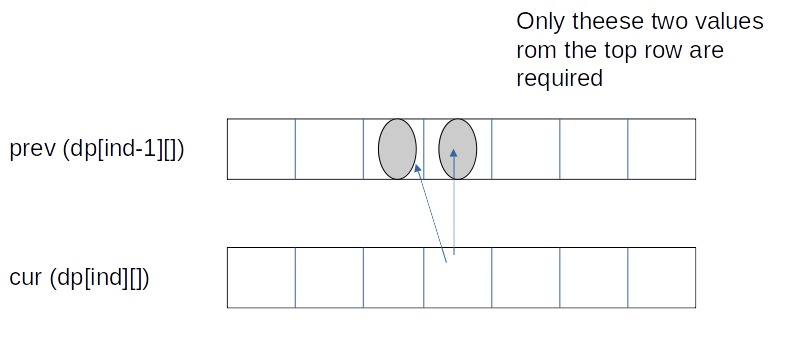If we need only two values from the prev row, there is no need to store an entire row. We can work a bit smarter.

We can use the cur row itself to store the required value in the following way:

• We take a single row ‘prev’.
• We initialize it to the base condition.
• Whenever we want to compute a value of the cell prev[j], we take the already existing value (prev[j] before new computation) and prev[j-1] (if required, in case of character match).
• We perform the above step on all the indexes.
• So we see how we can space optimize using a single row itself.

Code:

## C++ Code

``````#include <bits/stdc++.h>

using namespace std;

int prime = 1e9+7;

int subsequenceCounting(string &s1, string &s2, int n, int m) {
// Write your code here.

vector<int> prev(m+1,0);

prev=1;

for(int i=1;i<n+1;i++){
for(int j=m;j>=1;j--){ // Reverse direction

if(s1[i-1]==s2[j-1])
prev[j] = (prev[j-1] + prev[j])%prime;
else
prev[j] = prev[j]; //can omit this statemwnt
}
}

return prev[m];
}

int main() {

string s1 = "babgbag";
string s2 = "bag";

cout << "The Count of Distinct Subsequences is "<<
subsequenceCounting(s1,s2,s1.size(),s2.size());
}
``````

Output:

The Count of Distinct Subsequences is 5

Time Complexity: O(N*M)

Reason: There are two nested loops.

Space Complexity: O(M)

Reason: We are using an external array of size ‘M+1’ to store only one row.

## Java Code

``````import java.util.*;

class TUF{
static int prime =(int)(Math.pow(10,9)+7);

static int subsequenceCounting(String s1, String s2, int n, int m) {
// Write your code here.

int[] prev=new int[m+1];
prev=1;

for(int i=1;i<n+1;i++){
for(int j=m;j>=1;j--){ // Reverse direction

if(s1.charAt(i-1)==s2.charAt(j-1))
prev[j] = (prev[j-1] + prev[j])%prime;
else
prev[j] = prev[j]; //can omit this statemwnt
}
}

return prev[m];
}

public static void main(String args[]) {

String s1 = "babgbag";
String s2 = "bag";

System.out.println("The Count of Distinct Subsequences is "+
subsequenceCounting(s1,s2,s1.length(),s2.length()));
}
}
``````

Output:

The Count of Distinct Subsequences is 5

Time Complexity: O(N*M)

Reason: There are two nested loops.

Space Complexity: O(M)

Reason: We are using an external array of size ‘M+1’ to store only one row.

Special thanks to Anshuman Sharma for contributing to this article on takeUforward. If you also wish to share your knowledge with the takeUforward fam, please check out this article